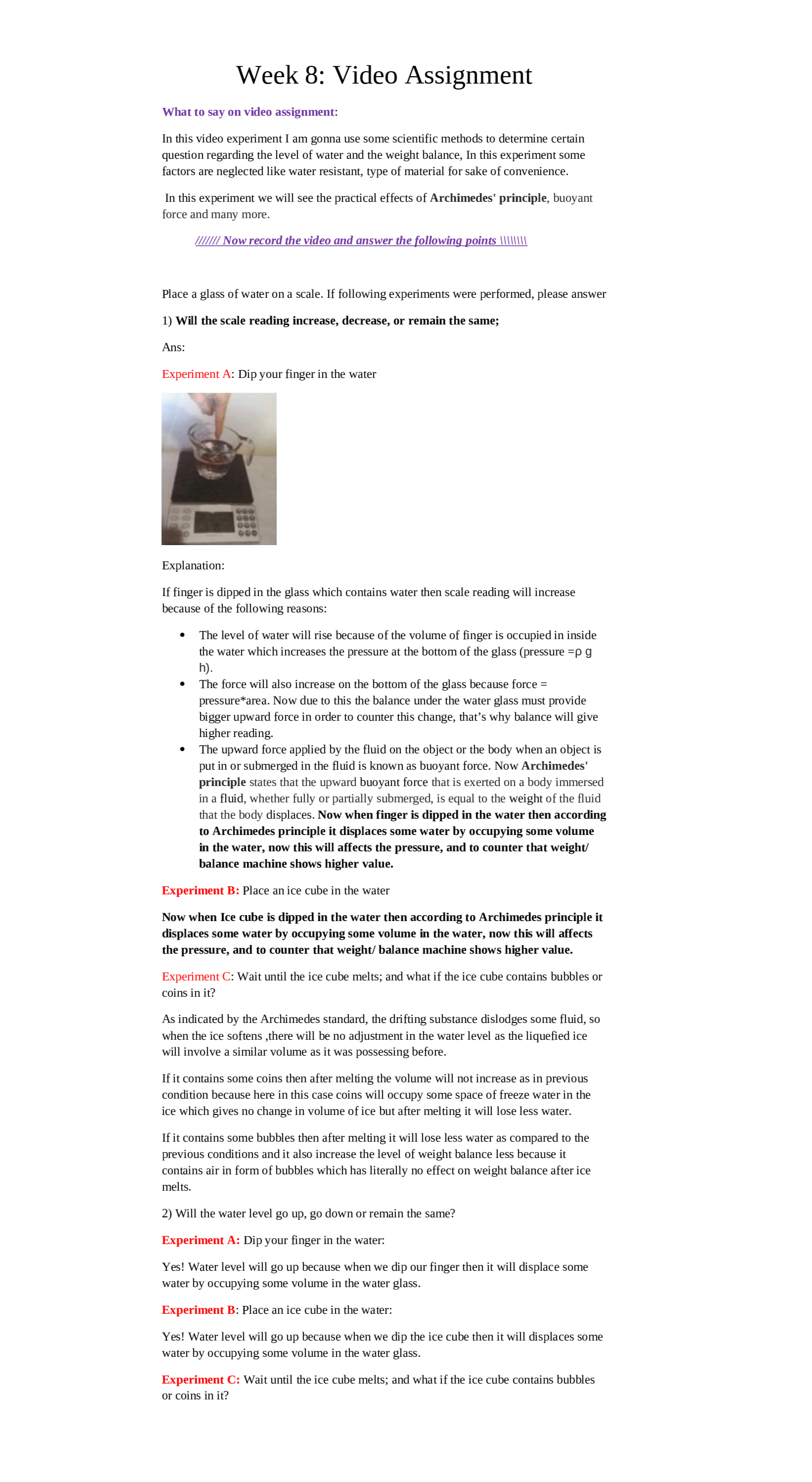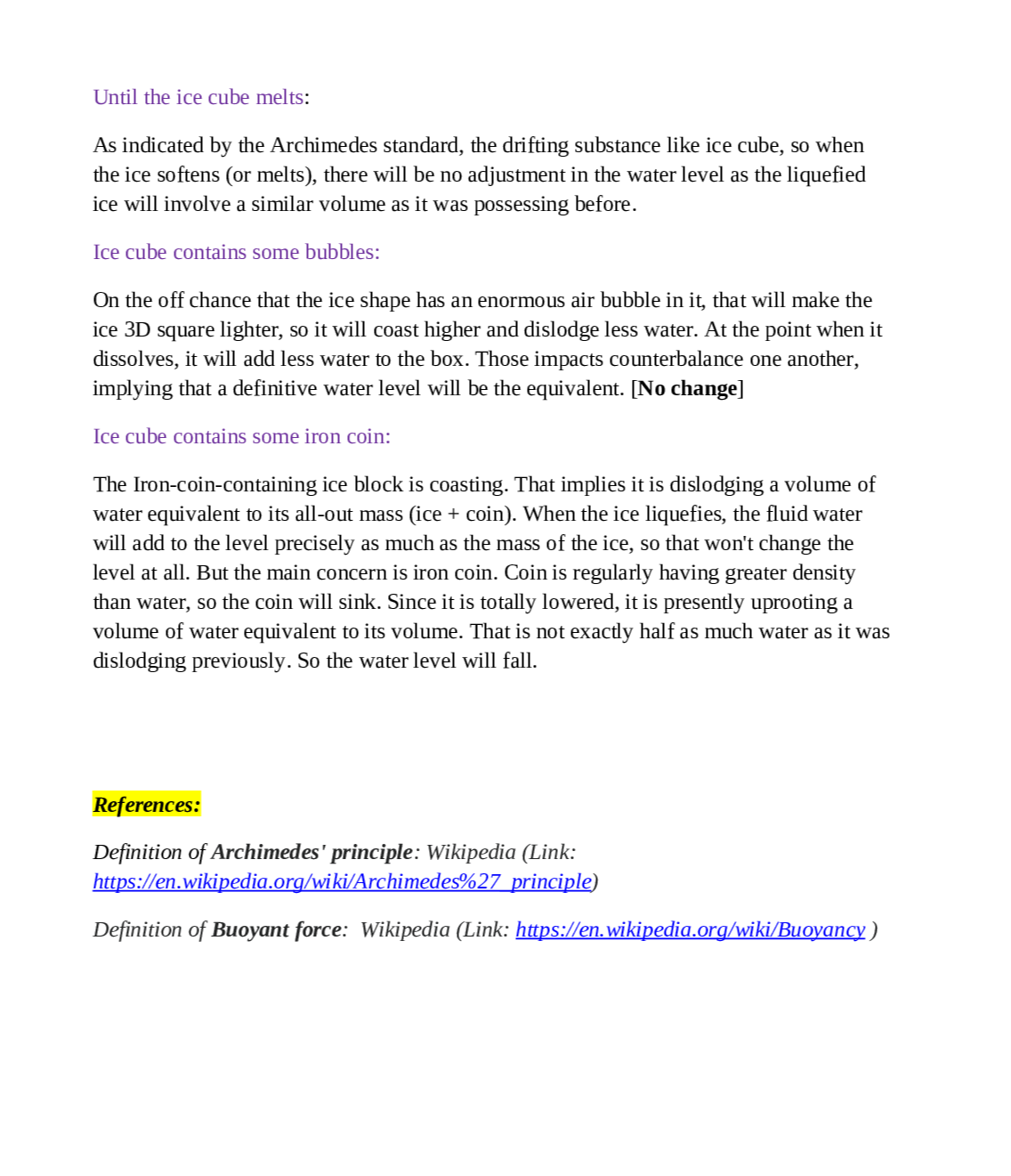Question

General Chemistry

Week 8: Video Assignment 3

Place a glass of water on a scale. If following experiments were performed, please answer 1) will the scale reading increase, decrease, or remain the same? 2) will the water level go up, go down or remain the same?

Experiment A: dip your finger in the water; Experiment B: place an ice cube in the water; Experiment C: wait until the ice cube melts; and what if the ice cube contains bubbles or coins in it?

Rules:

1) No portrait mode (if filming with a cell phone)2) Video must be less than three minute long 3) Must show your face - at least intermittently 4) Must voice narrate your explanation 5) Must hear your voice clearly 6) Video must be uploaded to YouTube

Tips:

Submission and Due Date:Submit your YouTube URL on Blackboard. Due 5:00 pm on Tuesday October 20, 2020.Verified### Question 45009General Chemistry

15.9 g of CaCO3 decomposed and 7.31 g of CaO are obtained what is the percent yield of CaO obtained
\mathrm{CaCO3}(\mathrm{s}) \rightarrow \mathrm{CaO}(\mathrm{s})+\mathrm{CO} 2(\mathrm{~g})

### Question 45008General Chemistry

(20). After completing a titration, you determined 0.52 mol of sodium hydroxide were required to reach the endpoint of sulfuric acid sample of unknown concentration. Your acid sample was 45 mL. What is the concentration of your acid? (Hint: is the reaction balanced?)
\mathrm{H} 2 \mathrm{SO} 4(\mathrm{aq})+\mathrm{NaOH}(\mathrm{aq}) \rightarrow \mathrm{Na2SO4}(\mathrm{aq})+\mathrm{H} 2 \mathrm{O}(1)

### Question 45007General Chemistry

(18). Find the mass of a metal object (in grams) that was heated to 105°C and placed in 27 g of water at 13°C The final temperature of the water is 40°C. The C water = 4.184 J/g°C and C metal = 0.184 J/g°C.

### Question 45006General Chemistry

(17). Determine the molarity of a solution formed by dissolving 48.9 g LiBr in enough water to yield a total solution volume of 750.0 mL.

### Question 45005General Chemistry

(12). A metal cube has an edge length of 2.44 cm and a mass of 16.28 g. Calculate the density of the metal.

### Question 44215General Chemistry

A lab technician had a sample of radioactive Americium. He knew it was one of the isotopeslisted in the table below but did not know which one. The sample originally contained 0.008g of the isotope, but 120 minutes later it contained 0.004 g. Use this information to answerthe following questions. (3 points)
A. How many half-lives have passed?
B. What is the half-life of the sample?
C. Which isotope did he have? (

### Question 44214General Chemistry

A. Name three kinds of radioactive decay and provide the symbol for each that is used to represent the product given off. (3 points)
\text { i. } a \text { - decay (1 point) }
\text { iii. Y - decay (1 point) }
\text { ii. } \beta \text { - decay (1 point) }
{ }_{1}^{2} \mathrm{H}+{ }_{1}^{2} \mathrm{H} \rightarrow \frac{3}{2} \mathrm{He}+{ }_{0}^{1} \mathrm{n}

### Question 44213General Chemistry

A. What two competing fundamental forces are at work inside the nucleus of an atom? (1point)
B. How can the ratio of number of protons to neutrons in the nucleus be used to predict thestability of a nucleus? (2 points)
C. How much energy would be released if 0.00023 kg of plutoniumthrough radioactive decay? (2 points)were converted to energy

### Question 44212General Chemistry

Use the emission spectra shown to answer the following questions (note the direction of thewavelength scale):
A. A high school student acquired an emission spectrum of an unknown sample. He knewthe unknown sample contained one of the elements, whose spectra are shown above. Theemission spectrum of his sample showed a strong emission at 610 nm but not at 480 nm.Which element did his unknown sample contain? (2 points)
B. Element A above has a strong emission around 670 nm. Does this emission line representa lower energy or higher energy transition than the emission line at 428 nm? Explain youranswer. (2 points)
C. Why does each element have its own unique emission spectrum? (2 points)

### Question 44211General Chemistry

A. Write a set of four possible quantum numbers for the circled electron. (There could bemore than one correct answer.) (4 points)
n =| =m, =II
B. The electron in a hydrogen atom can undergo a transition from n = 6 to n = 1, emitting aphoton with energy 2.11 x 10-18 J. (2 points)
i. What is the frequency of this transition? (1 point)
ii. How does this transition show that the energy of a photon is quantized? (1 point)
C. Why is it impossible for an electron to have the quantum numbers n = 3, | = 0, m, = 1, m, =
+\frac{1}{2} ?(2 \text { points })

### Submit query